# Cube-shaped box

Design the size of the smallest possible cube-shaped box where three types of 3cm, 5cm, 6cm small cubes could be stacked to make full use of the box space (each type of cube separately). Can you find out how many smallest cubes are in the box?

a =  30 cm
b =  1000

### Step-by-step explanation:

$b=\left(30\mathrm{/}3{\right)}^{3}=1000$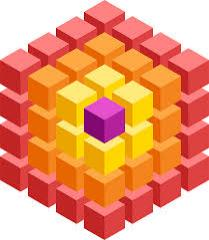Did you find an error or inaccuracy? Feel free to write us. Thank you!Tips to related online calculators
Do you want to calculate least common multiple two or more numbers?
Tip: Our volume units converter will help you with the conversion of volume units.

## Related math problems and questions:

• CubesCarol with cut bar 12 cm x 12 cm x 135 cm to the cubes. Find the sum of all the surfaces of the resulting cubes.
• Lcm 2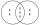Create the smallest possible number that is divisible by numbers 5,8,9,4,3
• Three cubesTwo cube-shaped boxes with edges a = 70 cm; b = 90 cm must be replaced by one cube-shaped box. What will be its edge?
• Two boxes-cubesTwo boxes cube with edges a=38 cm and b = 81 cm is to be replaced by one cube-shaped box (same overall volume). How long will be its edge?
• LCMCommon multiple of three numbers is 3276. One number is in this number 63 times, second 7 times, third 9 times. What are the numbers?
• Square roomWhat is the size of the smallest square room, which can pave with tiles with dimensions 55 cm and 45 cm? How many such tiles is needed?
• Minimum surfaceFind the length, breadth, and height of the cuboid-shaped box with a minimum surface area, into which 50 cuboid-shaped blocks, each with length, breadth, and height equal to 4 cm, 3 cm, and 2 cm respectively, can be packed.
• CherriesCherries in the bowl can be divided equally among 3 or 15 or 5 children. How many are the minimum cherries in the bowl?
• LCM of two numberFind the smallest multiple of 63 and 147
• The smallest numberWhat is the smallest number that can be divided by both 5 and 7
• Two gears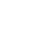A large gear will be used to turn a smaller gear. The large gear will make 75 revolutions per minute. The smaller gear must make 384 revolutions per minute. Find the smallest number of teeth each gear could have. [Hint: Use either GCF or LCM. ]
• A ropeA rope can be cut into equal length with no rope left over. The lengths can be 15cm,18cm or 25cm. What is the shortest possible length of the rope?
• Tailor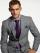From the rest of the cloth tailor could cut off either 3 m in men's suits without vest or 3.6 m with vest. What shortest possible length could the rest of the cloth have? How many suits a) without a vest b) with vest could make the tailor from the rest of
• The cubeThe cube has an edge of 25 cm. We cut it into small cubes of 5 cm long side. How many of these little ones left when we build a new cube of 20 cm in length?
• Around the flowerbed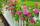Around a rectangular flowerbed with dimensions 5.25 m and 3.5 m are to be planted roses equally spaced so that the roses found in every corner of the flowerbed and consumed them as little as possible. a) At what distance are planted roses? b) How many ros
• LCMWhat is the least common multiple of 5, 50, 14?
• Matches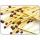George poured out of the box matches and composing them triangles and no match was left. Then he tries squares, hexagons and octagons and no match was left. How many matches must be at least in the box?